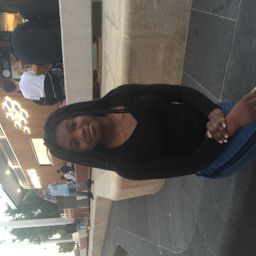Composition

### Discussion

You must be signed in to discuss.
##### Top Chemistry 101 Educators##### Morgan S.

University of Kentucky##### Jake R.

University of Toronto

### Video Transcript

art. So in this problem, we are looking at the hydrogen. Felix, they're looking at garden com phones involving the hay lights. So we have HF, uh, I ju fly. You have hydrogen chloride, hydrogen bromide and hydrogen. I indict. And we can also call these compounds. If there were solid surgeon Flora's enquired and stuff, but if they were to be equally is dissolved in water, this would be Hydrofluoric acid, hydrochloric Gaza and so on. And these are quite acidic compounds. One's dissolving in water. So, yes, So we are just here to find the mass percents of hydrogen. Yeah, mass percent. It's well what we're looking for, Sue. And we're not giving anything else other than the molecular formulas, which is, you know, and I So I'm going to created people here. So what is the first thing that we need to have determined first? Only just dreaming here is basically, um, mother mess. So mass percent just review is is the percent of is the percent ratio of one element, one mole of a limit in one more of compound basis. So we're working in one mole of compound, and it's like massive the element of mass of the compound. And since we're working in one mall are working, we're basically using mass. You were working in masses, so yeah, let me see mass percents. So we know that mass is equivalent to moles, times more mats and we're looking one mole, So beach in, basically use more masses here. That makes sense. Yes, always working with them. Also, this is always gonna be a one. So it's like the masses here will always equal minimus, so yes, So we're gonna take the molar mass of the compound and the molar mass of hydrogen and then divided. Yeah, it's a very straightforward and we would and we are basically using this formula over here. It's just we always are working in one more element when well off compound. And each element is just wrong of one mole of element in one mole of conference, one mole of hydrogen and one mole of cop out. So it's always everything's went. So in this case, we can use this if they were to be h two f. You know that that doesn't even possible. Then we would have toe, then multiply by a factor of two animals, so the molar mass of Haijun just to fill in this partner is basically 1.8 grams promote. I'm not gonna keep units cause working in mass. Technically. So yeah, working massive thing mass is equal toe molar mass. And we're gonna divide masses anyway to cancel out. So no unit. So it's the molar mass of margin. Just this and then mull mass of compound is this figure over here, multiply it, or in addition to the mullahs mats of each of these lines. So you can just look at your table and look for atomic mass, which is something more mass. So here we have that Arjun fluoride is equivalent to These is basically 20. That's the total mass of the compound. Each seal is 36.46 8 36.46 each. Beak are 80 points. 91 That shouldn't write little. Neither a 0.91 at h I. It's 1 27 i 05 So then it's always element over company Haijun over compound Arjun highlight to give us mass percent in this column. It's just double him this, I mean, and that basically gives us in percent so you would multiply by 100 5%. Basically for HF, 2.7 percent for it, Theo one point to percent Bridge B, R and H I always down one point 79%. So yes, it's quite small. And we can, like, conclude that as we increase the size of the highlights just by moving down the but column in the group the um Masterson of hydrogen is also going down and it appears to be in, like, half buy half approximately each time, So, yeah, there we have.McMaster University

#### Topics

Composition

##### Top Chemistry 101 Educators##### Morgan S.

University of Kentucky##### Jake R.

University of Toronto